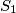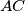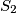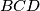### IMO Shortlist 2002 problem G1

Kvaliteta:
Avg: 2,0
Težina:
Avg: 6,0
Let$B$ be a point on a circle$S_1$, and let$A$ be a point distinct from$B$ on the tangent at$B$ to$S_1$. Let$C$ be a point not on$S_1$ such that the line segment$AC$ meets$S_1$ at two distinct points. Let$S_2$ be the circle touching$AC$ at$C$ and touching$S_1$ at a point$D$ on the opposite side of$AC$ from$B$. Prove that the circumcentre of triangle$BCD$ lies on the circumcircle of triangle$ABC$.
Izvor: Međunarodna matematička olimpijada, shortlist 2002